Octrees For Visibility Question submitted by (14 August 2001)Return to The Archives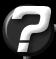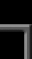Now, I've seen lot's and lot's of articles about octrees and about quadtrees, but I haven't found in any of them the actual code for determining if a quad/octree cell/a box in general is visible.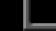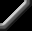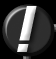The use of Octrees (including KD Trees and other variants) are simply a technique for hierarchically organizing your 3D geometry, they are not directly intended for use in visibility schemes. However, just like any data structure, they can serve many purposes, including visibility calculations.

There are a number of techniques that use Octrees for visibility determination. The most common is the user of hierarchical frustum culling. This works very well because even on today's fast 3D hardware, the overhead of performing hierarchical frustum culling is still less than rendering the geometry (at least, this is the case on today's 3D hardware.)

The process of hierarchical frustum culling is rather simple. A set of planes is generated that define the frusum (usually six planes, including hither & yon.) The Octree is traversed, and each node along the traversal is tested against an intersection with the view volume. From this test, one of three states is determined:
• 1. Completely outside the volume
• 2. Completely inside the volume
• 3. Partial inclusion in the volume
• Pseudocode for this traversal might look like this:

 ``` proc traverse(Node *n, Planes *list) { CODE = CodeToVolume(n, list); if (CODE == INSIDE) { // The entire branch is inside, render renderBranch(n); } else if (CODE == OUTSIDE) { // This node (and all children) are outside, skip return; } else // CODE == PARTIAL { // Render any polygons associated to this node renderNode(n); // Visit my children loop (i = 0 .. 8) { traverse(n->child[i], list); } } } ```

The CodeToVolume() routine isn't trivial, but it's also a far cry from complex. And with a few tricks, you can make it fairly efficient. Many implementations use the Cohen-Sutherland outcode technique for determining if a node is within the volume. This is tedious, because it requires that we test all points of each node against all planes.

Fortunately, there is a better way.

The idea is to test a maximum of two points (from the node) against each plane. During this process, we have an "early out" if a node is completely outside the volume's plane. For the sake of clarity, let's assume the planes that define our volume point inward toward the center of the volume. So the volume can be considered the intersection of all positive halfspaces defined by the set of planes.

On to the specifics...

Given a node and a plane, how do we best choose the point within the node for testing against a plane's halfspace? It depends on which halfspace we're testing. If we are testing for a node to be completely within the positive halfspace, then we must choose the point within the node that has the least potential of going positive. Therefore, if the chosen point is on the postive side, then so must all other points within the node.

In other words, when testing to see if a node is completely within the positive halfspace, we'll want a point from the node that is "most negative." The reverse is also true for testing against the negative halfspace.

The planes given may be arbitrary (but must define a convex volume.) Fortunately, because our Octree is orthogonal, this does not complicate the issue of choosing the best point. First, we can narrow our calculations down to just corner points of the node. Next, we'll use the plane's normal to determine the best corner. Remember, if we're testing for "completly positive" then we'll want the "most negative" corner, and vice versa. So in practice, we'll be using inverted normals.

Our node corner point will be the farthest point along the vector defined by the inverted normal. Also, remember this goes both ways:
• When testing for completely positive, we use the negative normal
• When testing for completely negative, we use the positive normal
• Confused yet? :)

This can be done with a simple index calculation and a table lookup. First, the table:

 ``` Point CornerOffsets[] = { Vector(-1, -1, -1), // - - - Vector( 1, -1, -1), // - - + Vector(-1, 1, -1), // - + - Vector( 1, 1, -1), // - + + Vector(-1, -1, 1), // + - - Vector( 1, -1, 1), // + - + Vector(-1, 1, 1), // + + - Vector( 1, 1, 1) // + + + }; ```

The order of this table is important. Notice the pattern in the indexing (the pattern is shown in the comments to the right.) This pattern defines a 3-bit value, where "-" is a zero-bit and "+" is a one-bit. If you know your binary conversions, you'll see that our -/+ patterns, when converted to 0/1 patterns, will defined the values 0-7, in order.

The code to calculate the index into this table (from a given normal) is as follows:

 ``` // Note that the input vector must already be negated when using this code for halfplane testsproc VectorToIndex(Vector v) { index = 0; if (v.z >= 0) index |= 1; if (v.y >= 0) index |= 2; if (v.x >= 0) index |= 4; return index; } ```

This index is then used to lookup a Vector from within the table. This vector, when multiplied by the radius of the node and added to the node's center point will return the correct corner point that is in the direction of the input vector.

We can now determine a corner point that is farthest in the direction of a vector. So let's get back to our volume inclusion test. We visit each plane in the volume and test the node for the negative halfspace. If the node is completely within the negative halfspace, the routine can quickly exit, knowing that the node is safely outside the volume. Otherwise, we'll need to test the node to see if it is completely within the positive halfspace. If not, then the node is bisected by this plane.

Note that the latter two cases (node is completely within the postive halfspace of a single plane, or bisected by that plane) is not our complete answer. Since there are multiple planes involved, a node may be within the positive halfspace of one plane, but within the negative halfspace of another. So when one of the two latter cases is detected, we must continue scanning our planes. The result looks something like this:

 ``` proc CodeToVolume(Node *n, Planes *list) { // Visit each plane once bool partialFlag = false; loop (i = 0 .. list[n]) { // Test against the negative halfspace first // // Note: we use the positive normal here Index = VectorToIndex(list[i]->normal); // Get the corner point of the node // // Note: The addition and multiplication operators // are component-wise operations negTestPoint = n->center + n->radius * CornerOffsets[Index]; // Test for completely within the negative halfspace of this plane // // If the halfplane test returns negative, the negTestPoint (as // well as the rest of the node) is within the negative halfspace, // so we can bail early. if (list[n].halfplane(negTestPoint) == -1) return OUTSIDE; // Test against the positive halfspace // // Note: we use the negative normal here Index = VectorToIndex(-list[i]->normal); // Get the corner point of the node // // Note: The addition and multiplication operators // are component-wise operations posTestPoint = n->center + n->radius * CornerOffsets[Index]; // Test for completely within the positive halfspace of this plane // // If the halfplane test returns negative, the posTestPoint is _not_ // completely within the positive halfspace, and therefore must be // bisected by the plane. if (list[n].halfplane(posTestPoint) == -1) partialFlag = true; } // At this point, the node must be either "partially included" // or completely included. The partial flag is used to determine // the result. if (partialFlag) return PARTIAL; return INSIDE; } ```

If you rip out all the comments, you'll see this routine is really quite short. One thing to note about this routine, is that a node may be returned as "partial" when in reality it is completely outside the volume. In practice I've seen that this is a fairly rare occurance (with a thousand nodes, I would expect only a half dozen on average.) I've tried adding code to produce a more exact result, but this actually slowed things down. This, of course, depends on the amount of CPU time an extra node costs you.

Aside from view frustum culling, Octrees have the potential for being fairly useful for occlusion culling. Many people have proposed a number of documents on the subject. One excellent example is the '93 paper "Hierarchical Z-Buffer Visibility" by Green, Kass & Miller [bib] . Later, in 96, Ned Greene revised the algorithm and published another paper titled "Hierarchical Polygon Tiling with Coverage Masks" [bib] .

There are also a number of other self-published articles and documents on the matter. However, in all of the cases I'm aware of, they have the drawback of being software driven. The problem is that in order to get proper visibility of a node, a set of occlusions must be given. The papers mentioned above use software rendering to build occlusion masks (either in the form of bit masks or z-buffers). The nodes are then tested against these masks by transforming, projecting and pixel-testing the extents of a node against the mask. Because of the software rendering involved, transforms must also be performed in software, so even if your renderer is extremely fast, the transforms alone tend to cause these techniques to have a high overhead.

If you were to use an estimation of a block of polygons (say, a bounding box) rather than the adding the individual polygons to the mask, you would get the wrong result. This is because the bounding box is solid, whereas the polygons contained within that box may not be solid (consider the back-facing polygons, for example.) So the group estimation must be a minimum bounds estimation. This becomes a view-dependent calculation that is more expensive than simply rendering the polygons into the mask.

One option is to use specific polygons as occluders. However, this causes the visibility to be greatly conservative. If a node is determined visible (because of the conservative nature) it's entire contents must be drawn (usually hundreds of polygons.) By increasing the tree resolution (and therefore reducing the number of polygons per node) you end up trading the efficiency of occlusions for the numerous tests of nodes against the mask.

The next logical step is to look to the hardware for rendering the mask. However, reading information back from the hardware can get rather expensive, depending on the API, drivers and particular chipset used.

If hardware were able to perform a z-test for you and return a boolean result, this would greatly reduce the problem because the z-buffer from the rendered polygons could be used as the mask for the occlusion test. However, this would require perfect front->back sorting, which is not a trivial matter when it comes to Octrees. Greene's '96 paper discusses this topic thoroughly and solves it by using an octree of BSP trees.

Don't let me discourage you from trying. I'm a firm believer that every challenge has a solution.Response provided by Paul Nettle